## A ball is thrown straight out at 80 feet per second from an upstairs window that’s 15 feet off the ground. Find the ball’s horizontal distan

Question

A ball is thrown straight out at 80 feet per second from an upstairs window that’s 15 feet off the ground. Find the ball’s horizontal distance from the window at the moment it strikes the ground.
a. 0.96825
b. Can’t be found
c. 77.46
d. 6.33
e. 212.23

in progress 0
3 months 2021-07-31T17:44:00+00:00 1 Answers 18 views 0

Step-by-step explanation:

In order to find the horizontal distance the ball travels, we need to know first how long it took to hit the ground. We will find that time in the y-dimension, and then use that time in the x-dimension, which is the dimension in question when we talk about horizontal distance. Here’s what we know in the y-dimension:

a = -32 ft/s/s

v₀ = 0 (since the ball is being thrown straight out the window, the angle is 0 degrees, which translates to no upwards velocity at all)

Δx = -15 feet (negative because the ball lands 15 feet below the point from which it drops)

t = ?? sec.

The equation we will use is the one for displacement:

Δx =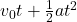and filling in: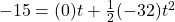which simplifies down to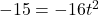so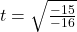so

t = .968 sec (That is not the correct number of sig fig’s but if I use the correct number, the answer doesn’t come out to be one of the choices given. So I deviate from the rules a bit here out of necessity.)

Now we use that time in the x-dimension. Here’s what we know in that dimension specifically:

a = 0 (acceleration in this dimension is always 0)

v₀ = 80 ft/sec

t = .968 sec

Δx = ?? feet

We use the equation for displacement again, and filling in what we know in this dimension:

Δx =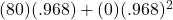and of course the portion of that after the plus sign goes to 0, leaving us with simply:

Δx = (80)(.968)

Δx = 77.46 feet